GFG App
Open AppBrowser
Continue

# Select row with maximum and minimum value in Pandas dataframe

Let’s see how can we select rows with maximum and minimum values in Pandas Dataframe with help of different examples using Python.

## Python3

 `# importing pandas and numpy` `import` `pandas as pd` `import` `numpy as np`   `# data of 2018 drivers world championship` `dict1 ``=` `{``'Driver'``: [``'Hamilton'``, ``'Vettel'``, ``'Raikkonen'``,` `                    ``'Verstappen'``, ``'Bottas'``, ``'Ricciardo'``,` `                    ``'Hulkenberg'``, ``'Perez'``, ``'Magnussen'``,` `                    ``'Sainz'``, ``'Alonso'``, ``'Ocon'``, ``'Leclerc'``,` `                    ``'Grosjean'``, ``'Gasly'``, ``'Vandoorne'``,` `                    ``'Ericsson'``, ``'Stroll'``, ``'Hartley'``, ``'Sirotkin'``],`   `         ``'Points'``: [``408``, ``320``, ``251``, ``249``, ``247``, ``170``, ``69``, ``62``, ``56``,` `                    ``53``, ``50``, ``49``, ``39``, ``37``, ``29``, ``12``, ``9``, ``6``, ``4``, ``1``],`   `         ``'Age'``: [``33``, ``31``, ``39``, ``21``, ``29``, ``29``, ``31``, ``28``, ``26``, ``24``, ``37``,` `                 ``22``, ``21``, ``32``, ``22``, ``26``, ``28``, ``20``, ``29``, ``23``]}`   `# creating dataframe using DataFrame constructor` `df ``=` `pd.DataFrame(dict1)` `print``(df.head(``10``))`

Output: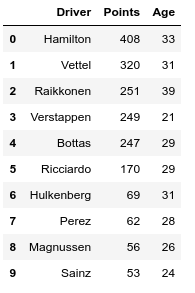## Select row with maximum value in Pandas Dataframe

Example 1: Shows max on Driver, Points, and Age columns.

## Python3

 `# creating dataframe using DataFrame constructor` `df ``=` `pd.DataFrame(dict1)`   `# the result shows max on` `# Driver, Points, Age columns.` `print``(df.``max``())`

Output: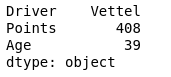Example 2: Who scored max points

## Python3

 `# creating dataframe using DataFrame constructor` `df ``=` `pd.DataFrame(dict1)`   `# Who scored more points ?` `print``(df[df.Points ``=``=` `df.Points.``max``()])`

Output: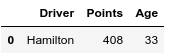Example 3: What is the maximum age

## Python3

 `# creating dataframe using DataFrame constructor` `df ``=` `pd.DataFrame(dict1)`   `# what is the maximum age ?` `print``(df.Age.``max``())`

Output:

`39`

Example 4: Which row has maximum age in the Dataframe | who is the oldest driver?

## Python3

 `# creating dataframe using DataFrame constructor` `df ``=` `pd.DataFrame(dict1)`   `# Which row has maximum age |` `# who is the oldest driver ?` `print``(df[df.Age ``=``=` `df.Age.``max``()])`

Output: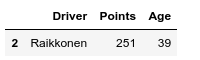## Select row with maximum value in Pandas Dataframe

Example 1: Shows min on Driver, Points, Age columns.

## Python3

 `# creating dataframe using DataFrame constructor` `df ``=` `pd.DataFrame(dict1)`   `# the result shows min on` `# Driver, Points, Age columns.` `print``(df.``min``())`

Output: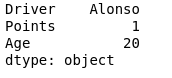Example 2: Who scored fewer points

## Python3

 `# creating dataframe using DataFrame constructor` `df ``=` `pd.DataFrame(dict1)`   `# Who scored less points ?` `print``(df[df.Points ``=``=` `df.Points.``min``()])`

Output: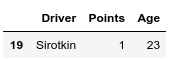Example 3: Which row has minimum age in the Dataframe who is the youngest driver

## Python3

 `# creating dataframe using DataFrame constructor` `df ``=` `pd.DataFrame(dict1)`   `# Which row has maximum age |` `# who is the youngest driver ?` `print``(df[df.Age ``=``=` `df.Age.``min``()])`

Output: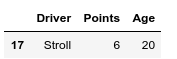My Personal Notes arrow_drop_up